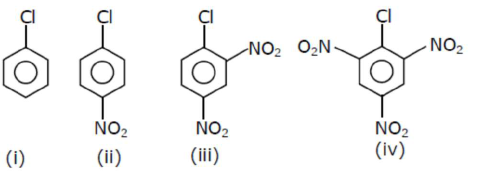# The correct order of the following compounds showing increasing tendency towards nucleophilic substitution reaction is :

Question:

The correct order of the following compounds showing increasing tendency towards nucleophilic substitution reaction is :1. $(i v)<(i)<(i i i)<(i i)$

2. $(\mathrm{i} v)<(\mathrm{i})<(\mathrm{ii})<(\mathrm{iii})$

3. $(i)<(i i)<(i i i)<(i v)$

4. (iv) $<$ (iii) $<$ (ii) $<$ (i)

Correct Option:

Solution:

Arrange the following metal complex/ compounds in the increasing order of spin only magnetic moment. Presume all the three, high spin system. (Atomic numbers $\mathrm{Ce}=58, \mathrm{Gd}=64$ and $\mathrm{Eu}=63 .)$

(a) $\left(\mathrm{NH}_{4}\right)_{2}\left[\mathrm{Ce}\left(\mathrm{NO}_{3}\right)_{6}\right]$

(b) $\mathrm{Gd}\left(\mathrm{NO}_{3}\right)_{3}$ and

(c) $\mathrm{Eu}\left(\mathrm{NO}_{3}\right)_{3}$

(1) $(b)<(a)<(c)$
(2) (c) $<(a)<(b)$
(3) $(a)<(b)<(c)$
(4) $(a)<(c)<(b)$